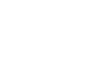what is simple pendulum? - letsdiskussANJU M.J.

Teacher | Posted | Education

# what is simple pendulum?

0
0

A simple pendulum can refer to one that acts like a simple pendulum despite its big amplitude, or one that behaves like a simple pendulum despite its huge amplitude. Remember that in a simple pendulum analysis, modest motions and very tiny angles are assumed.

OR

The simple pendulum is a mechanism made of an inextensible thread, attached to a support, the end of which carries a body of negligible size, which can travel freely.

When the instrument is stopped, it remains in a fixed position. The mass linked to the end of the wire oscillates about the equilibrium point when it is moved to a specific position.
As the body moves through the locations on the trajectory it follows, the pendulum moves at the same speed and acceleration.The simple pendulum is used in numerous investigations to evaluate the acceleration due to gravity.
Galileo Galileo is credited with being the first to notice the periodicity of pendulum motions and proposing the theory of pendulum oscillations.
A basic harmonic movement can be described as the movement of a pendulum that oscillates with a relatively tiny oscillation amplitude. The restoring force is the component of the weight force acting in the direction of motion, and it is equal to:  F=mgsenθ.

The pendulum movement is almost horizontal for very tiny angles, and the values of
sin θ ≈ θ the restoring force is nearly horizontal and can be calculated as follows:
F x =mgsenθ

We can write the displacement x from the equilibrium position as:  x=L.senθ

where L is the length of the pendulum string. Component F is:

F_x =-(m.g.x)/L
or
F x =-kx

Therefore, in the case of a pendulum of length L , the constant k holds: k=mg/L

Pendulum period
The periodic movement of a simple pendulum is defined as one that repeats in the same time intervals and may be determined using the period (T).

T=2π√(L/g)

Where,
T is the period, in seconds(s).
L is the length of the wire, in meters (m).
g is the acceleration of gravity, in (m/s 2 ).

The minimum time interval for the movement to occur may be determined using the period, and this formula must be used in small oscillations with angles less than 10º.

Restorative Strength

As gravity leads the pendulum to the lowest point, the restoring force (F) is responsible for causing it to return to its balanced state.
The restoring force is the horizontal component of the weight force, as determined by the position to which the body is directed on the pendulum. As a result, the formula is:

Energy Conservation in the PendulumTake a look at the illustration below. The letter A represents the equilibrium point of a basic pendulum. When you move it to the right, it will be in position B, and when you release it, it will be in position C.
The body at the wire's end acquires potential energy in position B. When you release it, the movement returns to position C, gaining kinetic energy but losing potential energy as the height decreases.
The potential energy is zero and the kinetic energy is highest when the body departs position B and arrives at location A.

Without taking into account air resistance, it may be assumed that the body in positions B and C achieve the same height and, as a result, the body has the same energy as it did at the start.
The overall mechanical energy of the body remains constant, indicating that this is a conservative system.  As a result, the mechanical energy will be the same at any point along the trajectory,.

0
0

@Student | Posted

A simple pendulum is a mechanical system that moves in oscillatory motion.  The motion is driven by a gravitational force and occurs generally in a vertical plane.  It contains a mass (m) supported by a massless and inextensible string. When the mass is pulled to one side, the pendulum is then set to motion and then the mass moves on the either side of its mean position.0
0